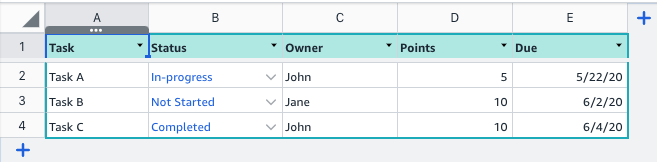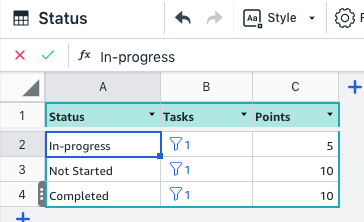# FILTER()

## Description

FILTER( ) filters a table or filter and returns a reference to rows matching the criteria when set as a source for a list. Multiple conditions can be combined using AND or OR.

## Syntax

`=FILTER(table, "condition", [condition_parameters, ...])`

Required

• Table/filter: A table or filter that returns a list of rows.

Optional

• Condition : Specify one or more Boolean formulas as a condition.

• Conditions can contain any supported functions
• Condition is expressed as a quoted string
• If the condition is omitted, all rows are returned
• Parameters: Conditions can be parameterized, like `Table[Column] = %.` In this case “%” will be replaced by the values provided in the condition parameters before the condition is evaluated.

## Examples### Specifying a condition

`=FILTER(Tasks, "Tasks[Status] = ""In-Progress""")`

Note: Since text values and conditions are wrapped in quotes, we use two double quotations in the filter condition.

### Combining multiple criteria

`=FILTER(Tasks,"Tasks[Owner]=""John"" AND Tasks[Due] < TODAY()+30")`

### Using parentheses to specify the order

`FILTER(Tasks,"Tasks[Owner]=""John"" AND (Tasks[Due] < TODAY()+30 OR Tasks[Status]=""Blocked"")“)`

### Parameterization

`FILTER(Tasks, "Tasks[Status] = % AND Tasks[Due] <= %", "In-Progress", TODAY()+30)`

A placeholder (%) can be used for substitution of parameters.

### Ordering the output

You can order the output using any column. By default, the ordering is performed in ascending order but you can use the keyword ASC (optional) or DESC to specify the order.

### Ordering multiple columns

`FILTER(Tasks, "Tasks[Status] = ""In-Progress"" ORDER BY Tasks[Status], Tasks[Due] Desc"`)`

When ordering multiple columns, the result is sorted in the order of the columns specified. Using the example table above, tasks will be ordered by status first and then order by date within the each status.

### Using filter outputs in other functions

`=SUM(Filter(Tasks, "Tasks[Milestone]=%", "Work")[Points])`

Filter output or columns can be used as an argument in functions like SUM, COUNT, COUNTA.

### Row count

`=ROWS(FILTER(...))`

To use number of rows returned by the filter in other arithmetic functions, use the ROWS() function

### Relating tables

`=FILTER(Tasks, "Tasks[Status]=[Status]")`

To get a list of tasks for each status stored in the table, you can use this format when `[Status]` is a column in the Status table.

### Using FILTER with rowlinks

`=FILTER(Tasks, "Tasks[Status]=THISROW()")`

When using with rowlinks, you can use also use THISROW() function.### Combining with existing filters

`=FILTER(FILTER(Tasks, "Tasks[Milestone]=%", "Work"), "Tasks[Status]=%", "Completed")`

Returns all tasks that match the milestone "Work" and have the status "Completed".